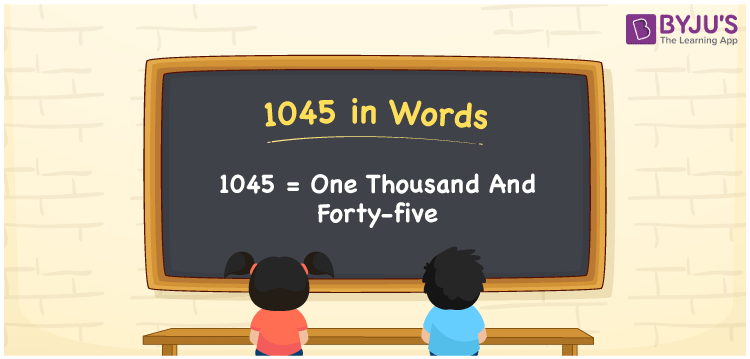# 1045 in Words

The numerical name for 1045 is One Thousand and Forty-Five. For instance, if you buy a makeup kit worth Rs. 1045, then you can say, “I bought a makeup kit worth Rupees One Thousand and Forty-Five”. 1045 is a cardinal number or counting number. The place value chart enables students to write the number names of any number effortlessly and express it in a better way. Learn the procedure of writing the number name for 1045 using a place value table and its expanded form in this article.

 1045 in Words One Thousand and Forty-Five One Thousand and Forty-Five in numerical form 1045

## 1045 in English Words

We generally express numbers in words using the letters of the English alphabet. Hence, the number 1045 in English can be written as One Thousand and Forty-Five.## How to Write 1045 in Words?

The number name for 1045 can be derived using a place value chart easily. As 1045 is a four-digit number, we need a table of 4 columns to depict its place value chart. The place value chart for 1045 is given below along with its expanded form.

 Thousands Hundreds Tens Ones 1 0 4 5

Therefore, we can write the expanded form as:

1 x Thousand + 0 x Hundred + 4 x Ten + 5 x One

= 1 x 1000 + 0 x 100 + 4 x 10 + 5 x 1

= 1000 + 0 + 40 + 5

= 1000 + 40 + 5

= 1045

= One Thousand and Forty-Five

Hence, 1045 in words is written as One Thousand and Forty-Five

Interesting way of writing 1045 in words

1 = One

10 = Ten

104 = One Hundred and Four

1045 = One Thousand and Forty-Five

Thus, the word form of the number 1045 is One Thousand and Forty-Five

1045 is a natural number that precedes 1046 and succeeds 1044

• 1045 in words – One Thousand and Forty-Five
• Is 1045 an odd number? – Yes
• Is 1045 an even number? – No
• Is 1045 a perfect square number? – No
• Is 1045 a perfect cube number? – No
• Is 1045 a prime number? – No
• Is 1045 a composite number? – Yes

## Frequently Asked Questions on 1045 in Words

Q1

### How do you write 1045 in words?

We can write 1045 in words as One Thousand and Forty-Five
Q2

### Simplify 1000 + 45, and express in words.

Simplifying 1000 + 45, we get 1045. Therefore, the number 1045 in words is One Thousand and Forty-Five.
Q3

### 1045 is an even number. True or False.

False, the number 1045 is not an even number.